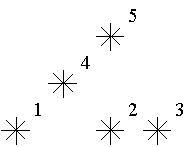# 深入理解树状数组C1 = A1
C2 = A1 + A2
C3 = A3
C4 = A1 + A2 + A3 + A4
C5 = A5
C6 = A5 + A6
C7 = A7
C8 = A1 + A2 + A3 + A4 + A5 + A6 + A7 + A8
...
C16 = A1 + A2 + A3 + A4 + A5 + A6 + A7 + A8 + A9 + A10 + A11 + A12 + A13 + A14 + A15 + A16

int lowerbit(int x)
{
return x&(x^(x–1));
}

int lowerbit(int x)
{
return x&-x;
}

step1:　令sum = 0，转第二步；
step2:　假如n <= 0，算法结束，返回sum值，否则sum = sum + Cn，转第三步；
step3: 令n = n – lowbit(n)，转第二步。

n = n – lowbit(n)这一步实际上等价于将n的二进制的最后一个1减去。而n的二进制里最多有log(n)个1，所以查询效率是log(n)的。

step1: 当i > n时，算法结束，否则转第二步；
step2: Ci = Ci + x， i = i + lowbit(i)转第一步。
i = i +lowbit(i)这个过程实际上也只是一个把末尾1补为0的过程。

lowbit(x):=x and -x;

lowbit(x)即为2^k的值。是不是很像一颗树？对，这就是为什么叫树状数组了～先看A图，a数组就是我们要维护和查询的数组，但是其实我们整个过程中根本用不到a数组，你可以把它当作一个摆设！c数组才是我们全程关心和操纵的重心。先由图来看看c数组的规则，其中c8 = c4+c6+c7+a8，c6 = c5+a6……先不必纠结怎么做到的，我们只要知道c数组的大致规则即可，很容易知道c8表示a1～a8的和，但是c6却是表示a5～a6的和，为什么会产生这样的区别的呢？或者说发明她的人为什么这样区别对待呢？答案是，这样会使操作更简单！看到这相信有些人就有些感觉了，为什么复杂度被lg了呢？可以看到，c8可以看作a1～a8的左半边和+右半边和，而其中左半边和是确定的c4，右半边其实也是同样的规则把a5～a8一分为二……继续下去都是一分为二直到不能分，可以看看B图。怎么样？是不是有点二分的味道了？对，说白了树状数组就是巧妙的利用了二分，她并不神秘，关键是她的巧妙！

她又是怎样做到不断的一分为二呢？说这个之前我先说个叫lowbit的东西，lowbit(k)就是把k的二进制的高位1全部清空，只留下最低位的1,比如10的二进制是1010,则lowbit(k)=lowbit(1010)=0010(2进制)，介于这个lowbit在下面会经常用到，这里给一个非常方便的实现方式，比较普遍的方法lowbit(k)=k&-k，这是位运算，我们知道一个数加一个负号是把这个数的二进制取反+1，如-10的二进制就是-1010=0101+1=0110，然后用1010&0110，答案就是0010了！明白了求解lowbit的方法就可以了，继续下面。介于下面讨论十进制已经没有意义（这个世界本来就是二进制的，人非要主观的构建一个十进制），下面所有的数没有特别说明都当作二进制。

上面那么多文字说lowbit，还没说它的用处呢，它就是为了联系a数组和c数组的！ck表示从ak开始往左连续求lowbit(k)个数的和，比如c=a+a，就是从110开始计算了0010个数的和，因为lowbit(0110)=0010，可以看到其实只有低位的1起作用，因为很显然可以写出c=a+a，这就为什么我们任何数都只关心它的lowbit，因为高位不起作用（基于我们的二分规则它必须如此！），除非除了高位其余位都是0，这时本身就是lowbit。

好吧，现在更新的次序都有了，可能又会产生新的疑问了：为什么它非要是这种关系啊？这就要追究到之前我们说c8可以看作a1～a8的左半边和+右半边和……的内容了，为什么c会影响到c而不会影响到c，这就是之前说的c的求解实际上是这样分段的区间 c~c 和区间c~c的和，数字太小，可能这样不太理解，在比如c会影响c，为什么呢？因为c可以看作0001～0100的和加上0101~1000的和，但是0101位置的数变化并会直接作用于c，因为它的尾部1不能一下在跳两级在产生两次高位1,是通过c间接影响的，但是，c却可以跳一级产生一次高位1。

可能上面说的你比较绕了，那么此时你只需注意：c的构成性质（其实是分组性质）决定了c只会直接影响c，而c只会直接影响，而下表之间的关系恰好是也必须是k +=lowbit(k)。此时我们就是写出跟新维护树的代码：

1 void add(int k,int num)
2 {
3        while(k<=n)
4         {
5             tree[k]+=num;
6             k+=k&-k;
7         }
8 }  

有了上面的基础，说求和就比较简单了。比如求0001～0110的和就直接c+c，分析方法与上面的恰好逆过来，而且写法也是逆过来的，具体就不累述了：

 1 int read(int k)//1~k的区间和
2 {
3        int sum=0;
4         while(k)
5         {
6             sum+=tree[k];
7             k-=k&-k;
8         }
9         return sum;
10 }  

POJ 2352

Stars
 Time Limit: 1000MS Memory Limit: 65536K Total Submissions: 45080 Accepted: 19567

Description

Astronomers often examine star maps where stars are represented by points on a plane and each star has Cartesian coordinates. Let the level of a star be an amount of the stars that are not higher and not to the right of the given star. Astronomers want to know the distribution of the levels of the stars.For example, look at the map shown on the figure above. Level of the star number 5 is equal to 3 (it's formed by three stars with a numbers 1, 2 and 4). And the levels of the stars numbered by 2 and 4 are 1. At this map there are only one star of the level 0, two stars of the level 1, one star of the level 2, and one star of the level 3.

You are to write a program that will count the amounts of the stars of each level on a given map.

Input

The first line of the input file contains a number of stars N (1<=N<=15000). The following N lines describe coordinates of stars (two integers X and Y per line separated by a space, 0<=X,Y<=32000). There can be only one star at one point of the plane. Stars are listed in ascending order of Y coordinate. Stars with equal Y coordinates are listed in ascending order of X coordinate.

Output

The output should contain N lines, one number per line. The first line contains amount of stars of the level 0, the second does amount of stars of the level 1 and so on, the last line contains amount of stars of the level N-1.

Sample Input

5
1 1
5 1
7 1
3 3
5 5

Sample Output

1
2
1
1
0

Hint

This problem has huge input data,use scanf() instead of cin to read data to avoid time limit exceed.

Source

就是求每个小星星左小角的星星的个数。坐标按照Y升序，Y相同X升序的顺序给出

 1 #include <stdio.h>
2 #include <string.h>
3 const int MAXN=32005;
4 const int MINN=15005;
5 int tree[MAXN];//下标为横坐标
6 int level[MINN];//下标为等级数
7 /*int lowerbit(int x)
8 {
9     return x&-x;
10 }*/
11 void add(int k,int num)
12 {
13     while(k<=MAXN)
14     {
15         tree[k]+=num;
16         k+=k&-k;
17     }
18 }
19 int read(int k)//1~k的区间和
20 {
21     int sum=0;
22     while(k)
23     {
24         sum+=tree[k];
25         k-=k&-k;
26     }
27     return sum;
28 }
29 int main()
30 {
31     int n,x,y,i;
32     memset(tree,0,sizeof(tree));
33     memset(level,0,sizeof(level));
34     while(scanf("%d",&n)!=EOF)
35     {
36         for(i=1;i<=n;i++)
37         {
38             scanf("%d%d",&x,&y);
40             level[temp]++;
47 }